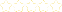• > Abbe parham
• > Integers
+# Integers

Owner: Abbe parham
##### Description:
Math 7 unit 5 This unit includes the following topics; compare & order integers, absolute value, add, subtract, multiply and divide integers.

### Your Quiz Points in this Playlist

Total Possible
0
13 Tutorials in this Playlist

#### Integers and Absolute Value

Author: Anna Hunter
Rating:(5)

Watch the video. Then answer the "check your progress questions" in your math journal. Show all work.

#### Absolute Value

Author: Dr. C
Rating:(25)
This tutorial has a quiz
•• -

Watch the video, then complete the exit ticket quiz in the upper right-hand corner.

#### Real Numbers

Author: Parmanand Jagnandan
Rating:(1)

Watch the first 2 videos and take notes in your math journal. Your notes should be on natural numbers and positive & negative numbers.

#### Convert, Compare, and Order Rational Numbers and Integers

Author: Danny Beaver
Rating:(6)

Watch the video "compare & order rational numbers and integers". QUESTION: . Is an integer a rational number? Why or why not? explain and give an example in your explanation.

#### Math 7 :: Unit 1 : Lesson 3 : Adding Integers

Author: Lloyd Murphy
Rating:(8)

Watch both videos. Take notes. Then, answer the following questions. . 12 + 4 = . 12 + (-4) = . (-12) + 4 = . (-12) + (-4) = . Why are the answers not the same. Make sure you can explain.

Author: Vilencia Bowman
Rating:(9)

Watch each video. Then in your math journal complete the worksheet and the summary question at the end.

Author: Kim Chavis
Rating:(4)

Watch the video. Then complete the question and answer section below.

#### Multiplying Integers

Author: Annette Booth
Rating:(4)

#### Dividing positive and negative numbers

Author: Renee Bosco
Rating:(4)

#### 7th grade 1-3 Multiplying and Dividing Integers

Author: Scot Sinclair
Rating:(11)

Watch the video at the bottom of the page. Then complete and submit the answers to the questions.